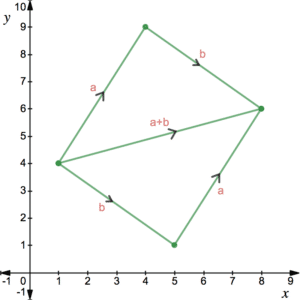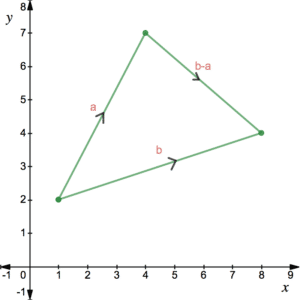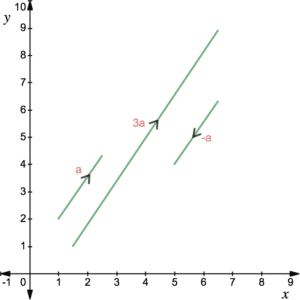# Vector Arithmetic

Addition, subtraction and scalar multiplication of vectors is very straight forward. Vector multiplication and vector division, however, do not make sense and therefore do not have a definition.To add two vectors together, one simply adds together the corresponding components. For example:

$\left(\begin{array}{c}3\\5\end{array}\right)+\left(\begin{array}{c}4\\-3\end{array}\right)=\left(\begin{array}{c}7\\2\end{array}\right)$

The vector that results from applying one vector followed by another by adding, i.e. ${\bf a}+{\bf b}$ is the vector that points directly from the start point to the finish point. Applying the vectors the other way round, i.e. ${\bf b}+{\bf a}$, also results in the same resultant vector. This is known as the parallelogram law of vector addition. See figure.

This may also be written as $\overrightarrow{AB}+\overrightarrow{BC}=\overrightarrow{AC}$ if considering vectors between points $A$, $B$ and $C$.

## Vector SubtractionAs you might expect, vector subtraction is achieved by subtracting the corresponding components of the given vectors. For example:

$\left(\begin{array}{c}3\\-5\end{array}\right)-\left(\begin{array}{c}-4\\2\end{array}\right)=\left(\begin{array}{c}7\\-7\end{array}\right)$. Rather than starting from a point and moving along two vectors one after the other, consider two vectors starting from the same point, say ${\bf a}$ and ${\bf b}$. The vector that results from subtracting ${\bf a}$ from ${\bf b}$ is the one that points directly from the end of ${\bf a}$ to the end of ${\bf b}$. See figure. This is known as the triangle law of vector addition. It can be thought of as starting at the end of ${\bf a}$, moving backwards along ${\bf a}$ and then forwards along ${\bf b}$ ($-{\bf a}+{\bf b}$) but written as (${\bf b}-{\bf a}$). See Position Vectors for more on this.

## Scalar Multiplication of VectorsThere is no definition for multiplying vectors together but we can multiply or divide vectors by a scalar (ie a single number). For example,

$2\left(\begin{array}{c}-1\\6\end{array}\right)=\left(\begin{array}{c}-2\\12\end{array}\right)$  or $-\frac{1}{3}\left(\begin{array}{c}3\\-2\end{array}\right)=\left(\begin{array}{c}-1\\\frac{2}{3}\end{array}\right)$

As we can see from the diagram, scalar multiples of vectors are all parallel.

Since scalar multiplication and vector addition is possible, it follows that any vector can be expressed as a linear combination of the standard unit vectors. For example,

$\left(\begin{array}{c}7\\-5\end{array}\right)=\left(\begin{array}{c}7\\0\end{array}\right)-\left(\begin{array}{c}0\\5\end{array}\right)=7\left(\begin{array}{c}1\\0\end{array}\right)-5\left(\begin{array}{c}0\\1\end{array}\right)=7{\bf i}-5{\bf j}$

This is useful when writing vectors on a single line rather than stacked horizontally.

## Examples

Consider the following vectors:

${\bf a}=\left(\begin{array}{c}-1\\3\end{array}\right)$,     ${\bf b}=\left(\begin{array}{c}8\\5\end{array}\right)$,    ${\bf c}=\left(\begin{array}{c}4\\-2\end{array}\right)$.

The vector $6{\bf a}+4{\bf b}-9{\bf c}$ is parallel to the vector $5{\bf i}+k{\bf j}$. Find the value of $k$.

The parallelogram $ABCD$ has 3 of its vertices at $A(-2,0)$, $B(3,2)$ and $C(4,-2)$. Find the coordinates of point $D$.